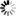Welcome to Central Library, SUST
Normal view

# Concise Computer Mathematics [electronic resource] : Tutorials on Theory and Problems / by Ovidiu Bagdasar.

Material type:TextPublisher: Cham : Springer International Publishing : Imprint: Springer, 2013Description: XII, 109 p. 17 illus. online resourceContent type:
• text
Media type:
• computer
Carrier type:
• online resource
ISBN:
• 9783319017518
Additional physical formats: Printed edition:: No titleDDC classification:
• 004.0151 23
LOC classification:
• QA76.9.M35
Online resources:
Contents:
Sets and Numbers -- Relations and Databases -- Functions -- Boolean Algebra, Logic and Quantifiers -- Normal Forms, Proof and Argument -- Vectors and Complex Numbers -- Matrices and Applications -- Matrix Transformations for Computer Graphics -- Elements of Graph Theory -- Elements of Number Theory and Cryptography -- Elements of Calculus -- Elementary Numerical Methods.
Summary: Adapted from a modular undergraduate course on computational mathematics, Concise Computer Mathematics delivers an easily accessible, self-contained introduction to the basic notions of mathematics necessary for a computer science degree. The text reflects the need to quickly introduce students from a variety of educational backgrounds to a number of essential mathematical concepts. The material is divided into four units: discrete mathematics (sets, relations, functions), logic (Boolean types, truth tables, proofs), linear algebra (vectors, matrices and graphics), and special topics (graph theory, number theory, basic elements of calculus). The chapters contain a brief theoretical presentation of the topic, followed by a selection of problems (which are direct applications of the theory) and additional supplementary problems (which may require a bit more work). Each chapter ends with answers or worked solutions for all of the problems.
Tags from this library: No tags from this library for this title.
Star ratingsAverage rating: 0.0 (0 votes)
No physical items for this record

Sets and Numbers -- Relations and Databases -- Functions -- Boolean Algebra, Logic and Quantifiers -- Normal Forms, Proof and Argument -- Vectors and Complex Numbers -- Matrices and Applications -- Matrix Transformations for Computer Graphics -- Elements of Graph Theory -- Elements of Number Theory and Cryptography -- Elements of Calculus -- Elementary Numerical Methods.

Adapted from a modular undergraduate course on computational mathematics, Concise Computer Mathematics delivers an easily accessible, self-contained introduction to the basic notions of mathematics necessary for a computer science degree. The text reflects the need to quickly introduce students from a variety of educational backgrounds to a number of essential mathematical concepts. The material is divided into four units: discrete mathematics (sets, relations, functions), logic (Boolean types, truth tables, proofs), linear algebra (vectors, matrices and graphics), and special topics (graph theory, number theory, basic elements of calculus). The chapters contain a brief theoretical presentation of the topic, followed by a selection of problems (which are direct applications of the theory) and additional supplementary problems (which may require a bit more work). Each chapter ends with answers or worked solutions for all of the problems.

There are no comments on this title.